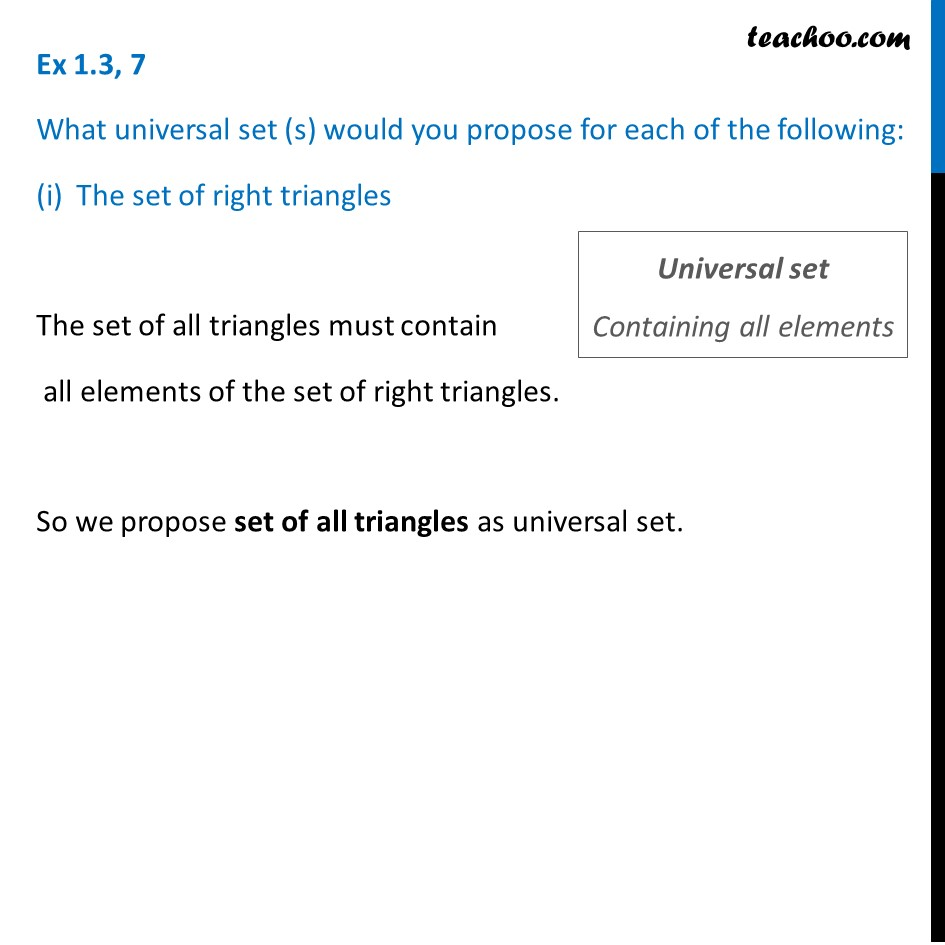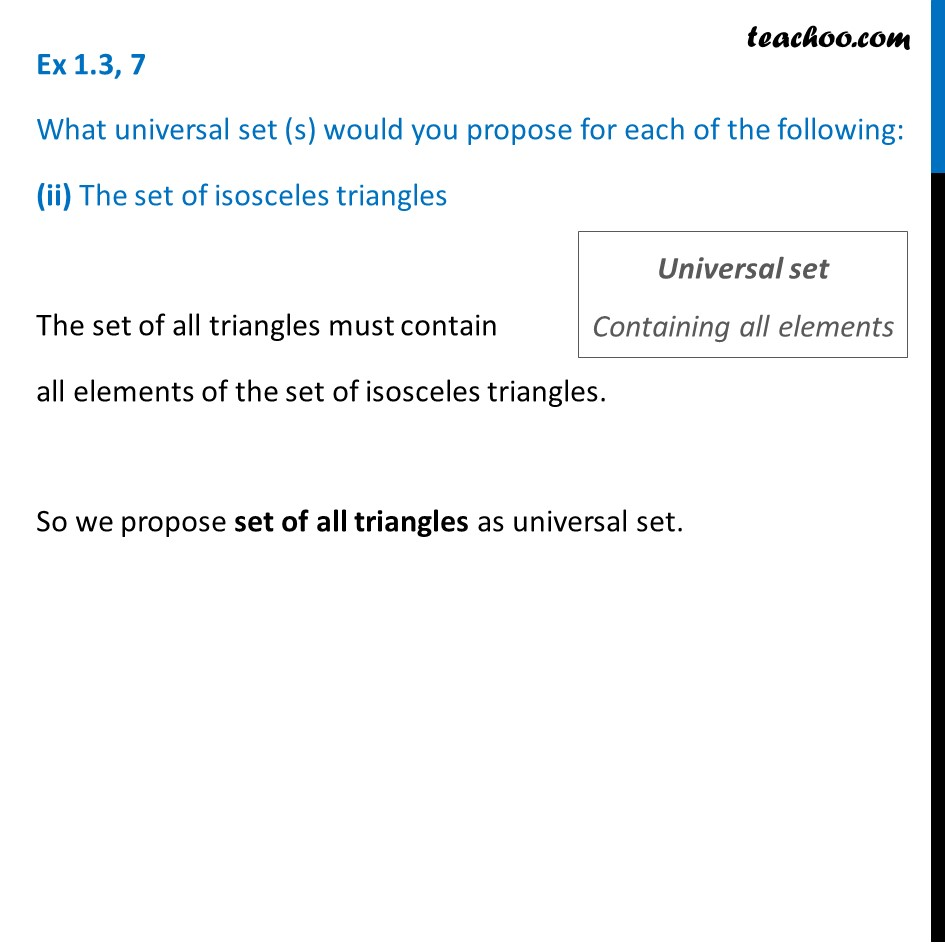Ex 1.3

Chapter 1 Class 11 Sets
Serial order wiseLearn in your speed, with individual attention - Teachoo Maths 1-on-1 Class

### Transcript

Ex 1.3, 7 What universal set (s) would you propose for each of the following: The set of right triangles The set of all triangles must contain all elements of the set of right triangles. So we propose set of all triangles as universal set. Ex 1.3, 7 What universal set (s) would you propose for each of the following: (ii) The set of isosceles triangles The set of all triangles must contain all elements of the set of isosceles triangles. So we propose set of all triangles as universal set.